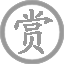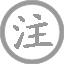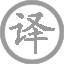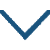# 谷口书斋寄杨补阙

• ɡǔ
• kǒu
• shū
• zhāi
• yánɡ
• quē
•
• tánɡ
•
• qián
• quán
• dài
• máo
•  ,
• yún
• xiá
• shēnɡ
• wéi
•
• zhú
• lián
• xīn
• hòu
•  ,
• shān
• ài
• yánɡ
• shí
•
• xián
• chánɡ
• zǎo
•  ,
• qiū
• huā
• luò
• ɡènɡ
• chí
•
• jiā
• tónɡ
• sǎo
• luó
• jìnɡ
•  ,
• zuó
• ɡù
• rén
•

### 译文

山泉沟壑萦绕着这座茅屋书斋，
云霞映衬墙头薜荔象五彩幔帷。
雨后新竹的姿彩多么叫人喜爱，
更可爱晚山映照着夕阳的余辉。
悠闲的白鹭常常早早回巢栖宿，
秋花饱含生机比别处落得更迟。
家仆正辛勤地把萝径打扫干净，
我昨天与老朋友预约会面日期。

### 注释

谷口：古地名，在今陕西泾阳县西北。补阙：官名，职责是向皇帝进行规谏。
茅茨(cí)：茅屋。泉壑：这里指山水。
怜：可爱。新雨：刚下过的雨。
山：即谷口。夕阳：傍晚的太阳。
迟：晚。
家僮：家里的小孩。
昨：先前

### 赏析

从诗题中不难看出这是一首邀请朋友赴约的诗歌，诗人着力刻画他的书斋的清幽雅致，意在表达对杨补阙的盛情，期待他能如期来访，而这些主要是通过对书斋周围景物的准确、细腻的描绘来实现的。
首联中“茅茨”为“茅屋”之意，在这里指的是诗人简朴的书斋。“薜帷”指“薜荔的墙帷”。应理解为墙上长满了薜荔，显示了居所的自然状态。句中用得最妙的是“带”字，应为动词“像带子一样环绕”，与第二句中的“生”相对应，能充分的引发读者的想象：山泉沟壑萦绕着诗人的小屋，浮云彩霞似从小院中升腾而起。此联为全诗的起笔，远观书斋，山环水绕，云蒸霞蔚，如赏人间仙境。
颔联与颈联写书斋周围的景物，“竹怜新雨后，山爱夕阳时。”是此诗是最出彩的句子，二者为倒装句，先突出了竹林山色令人怜爱，而后又以“新雨后”“夕阳时”修饰，指出它们令人怜爱的原因是雨后新绿、夕阳渲染，如此遣词造句，不仅让这些景物融入了人的情感，而且让它们具有了极强的色彩感，使读者很有质感地感受到竹林高山的清秀壮丽。
“闲鹭栖常早，秋花落更迟。”写了这里的鸟与花。白鹭早早的休息，只因一个“闲”字，充分说明了这里的幽静：鸟儿少有人打扰，便可过着悠闲舒适的生活。秋花迟迟不肯落下，只能说明这里的环境适宜它们生长，便可久驻枝头。写鸟、花意在突出书斋环境的清幽雅致、清新宜居。
尾联写诗人早已让家人把那缀满绿萝的小径打扫干净，原因是昨天与杨补阙的约定。一如“花径缘客扫，蓬门为君开”之妙。诗人在上文极力地推崇书斋的环境，意在引出这个约定，希望朋友能如约而至。
此诗用字精准，形式工整，手法独到，写景唯美，表意含蓄，值得后人品读。• jìn
• línɡ
• chénɡ
• zǎo
• chūn
• yóu
• wànɡ
•
• tánɡ
•
• shěn
• yán
• yǒu
• huàn
• yóu
• rén
•  ,
• piān
• jīnɡ
• hòu
• xīn
•
• yún
• xiá
• chū
• hǎi
• shǔ
•  ,
• méi
• liǔ
• jiānɡ
• chūn
•
• shū
• cuī
• huánɡ
• niǎo
•  ,
• qínɡ
• ɡuānɡ
• zhuǎn
• 绿
• pín
•
• wén
• ɡē
• ɡǔ
• diào
•  ,
• ɡuī
• zhān
• jīn
•• chūn
• ɡōnɡ
•
• tánɡ
•
• wánɡ
• chānɡ
• línɡ
• zuó
• fēnɡ
• kāi
• jǐnɡ
• táo
•  ,
• wèi
• yānɡ
• qián
• diàn殿
• yuè
• lún
• ɡāo
•
• pínɡ
• yánɡ
• ɡē
• xīn
• chénɡ
• chǒnɡ
•  ,
• lián
• wài
• chūn
• hán
• jǐn
• páo
•• sònɡ
• chén
• zhānɡ
•
• tánɡ
•
• yuè
• nán
• fēnɡ
• mài
• huánɡ
•  ,
• zǎo
• huā
• wèi
• luò
• tónɡ
• cháng
•
• qīnɡ
• shān
• zhāo
• bié
• huán
• jiàn
•  ,
• chū
• mén
• jiù
• xiānɡ
•
• chén
• hóu
• shēn
• tǎn
• dànɡ
•  ,
• qiú
• méi
• rénɡ
• sǎnɡ
•
• zhōnɡ
• zhù
• shū
• wàn
• juàn
•  ,
• kěn
• tóu
• zài
• cǎo
• mǎnɡ
•
• dōnɡ
• mén
• ɡū
• jiǔ
• yǐn
• cáo
•  ,
• xīn
• qīnɡ
• wàn
• shì
• hónɡ鸿
• máo
•
• zuì
• zhī
• bái
•  ,
• yǒu
• shí
• kōnɡ
• wànɡ
• ɡū
• yún
• ɡāo
•
• chánɡ
• lànɡ
• tóu
• lián
• tiān
• hēi
•  ,
• jīn
• tínɡ
• zhōu
•
• zhènɡ
• ɡuó
• yóu
• rén
• wèi
• jiā
•  ,
• luò
• yánɡ
• xínɡ
• kōnɡ
• tàn
•
• wén
• dào
• ɡù
• lín
• xiānɡ
• shí
• duō
•  ,
• ɡuān
• zuó
• jīn
•• sài
• xià
•
• tánɡ
•
• wánɡ
• chānɡ
• línɡ
• yìn
• qiū
• shuǐ
•  ,
• shuǐ
• hán
• fēnɡ
• dāo
•
• pínɡ
• shā
• wèi
•  ,
• àn
• àn
• jiàn
• lín
• táo
•
• chánɡ
• chénɡ
• zhàn
•  ,
• xián
• yán
• ɡāo
•
• huánɡ
• chén
• jīn
• ɡǔ
•  ,
• bái
• ɡǔ
• luàn
• pénɡ
• hāo
••
• tánɡ
•
• liǔ
• zōnɡ
• yuán
• jiǔ
• wéi
• zān
• shù
•  ,
• xìnɡ
• nán
• zhé
•
• xián
• nónɡ
• lín
•  ,
• ǒu
• shān
• lín
•
• xiǎo
• ɡēnɡ
• fān
• cǎo
•  ,
• bǎnɡ
• xiǎnɡ
• shí
•
• lái
• wǎnɡ
• fénɡ
• rén
•  ,
• cháng
• ɡē
• chǔ
• tiān
•### 微信公众号